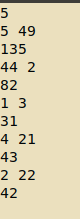# WA in SWPDGT

prolem link:CodeChef: Practical coding for everyone
my code:
#include <bits/stdc++.h>
using namespace std;
int main()
{
long long int i,j,k,l,x,y,z,t,m,n;
cin>>t;
while(t–)
{
cin>>m>>n;
x=m%10;
y=n%10;
i=m/10;
j=n/10;
k=x-j;
l=y-i;
if(k<=0&&l<=0)
cout<<m+n<<endl;
else if(k>l)
{
m=(m/10)10+j;
n=x
10+y;
cout<<m+n<<endl;
}
else
{
m=y*10+x;
n=(n/10)*10+i;
cout<<m+n<<endl;
}
}
}YOU CODE IS NOT WORKING ON SINGLE DIGIT NUMBER

2 Likes

thanks brother .Can i have your code?

1 Like

He’s a 5 star coder and Division 1 doesn’t have the first 2 questions of division 2.
If you want you can have my code but it’s kind of messy. You should probably check your code for test cases like: 1 99 and single digit numbers

1 Like

what should be output for 1 99 ??

#include <bits/stdc++.h>
using namespace std;
int main() {
// ios::sync_with_stdio(false); cin.tie(0); cout.tie(0);
// cout.precision(8);
// cout << fixed;
int T,t=0;
cin>>T;
while(t++<T)
{
// cout<<“Case #”<<t<<": ";
int a,b,s1=0,s2=0;
cin>>a>>b;
int a1={0},b1={0};
a1=a%10;
a1=(a/10)%10;
b1=b%10;
b1=(b/10)%10;
if(a1<b1)
{
s1+=b1*10+a1;
s1+=b1*10+a1;
}
if(b1<a1)
{
s2+=a1*10+b1;
s2+=a1*10+b1;
}
cout<<max(s1,s2)<<endl;
}

``````return 0;
``````

}
brother my code is giving values as expected by you but still giving wa

sorry bro.I am new in codechef .Now my code get ac.Now i will see your code.because i use too much unnecessary condition.

1 Like

Bro, the access is denied can you put the code or screenshot

100 should be the output.

1 Like

What went wrong in my code ?

``````#include <bits/stdc++.h>
using namespace std;

#define mod             1000000007
#define rep(i,n)        for (i = 0; i < n; ++i)
#define REP(i,k,n)      for (i = k; i <= n; ++i)
#define REPR(i,k,n)     for (i = k; i >= n; --i)
#define w(x)            int x; cin>>x; while(x--)
#define mk(arr,n,type)  type *arr=new type[n];
#define inrange(i, a, b) ((i >= min(a, b)) && (i <= max(a, b)))

template<typename T>
void printList(std::initializer_list<T> list){
for(const auto &val : list)
cout << val << " ";
cout << "\n";
}

void solve2(){
int a,b;
cin >> a >> b;
int x1 = 0, x2 = 0, x3 = 0, x4 = 0;
x1 = a % 10;
if(a > 9){
x2 = (a / 10) % 10;
}
x3 = b % 10;
if(b > 9){
x4 = (b / 10) % 10;
}
if(x2 == 0 && x4 == 0){
cout << a + b << "\n";
return;
}
// x2 x1
// x4 x3
int n1 = x3 * 10 + x1;
int n2 = x4 * 10 + x2;

int n3 = x1 * 10 + x3;
int n4 = x2 * 10 + x4;

int n5 = x4 * 10 + x1;
int n6 = x2 * 10 + x3;
cout << max(n1 + n2, max(n3 + n4, max(n5 + n6, a+b)));
cout << "\n";
}

int main(){
ios_base::sync_with_stdio(false);
cin.tie(0);
cout.tie(0);
#ifndef ONLINE_JUDGE
freopen("input.txt", "r", stdin);
freopen("output.txt", "w", stdout);
#endif

return 0;
}``````

My ac code:
#include <bits/stdc++.h>
using namespace std;
int main()
{
long long int i,j,k,l,x,y,z,t,m,n;
int a;
cin>>t;
while(t–)
{
cin>>n>>m;
for(i=0;i<n;i++)
cin>>a[i];
sort(a,a+n);
l=0;
z=n-1;
while(l<=z)
{
k=(l+z)/2;
x=0;
for(i=0;i<n;i++)
{
if(i>k)
x=x+a[k];
else
x=x+a[i];
}
if(x==m)
{
cout<<a[k]<<endl;
break;
}
else if(x>m)
z=k-1;
else
l=k+1;
}
if(l>z)
cout<<"-1"<<endl;
}
}
Hope it will help someone.

thankshttps://www.codechef.com/viewsolution/30841233

i think you didn’t call the function solve and no test cases option are there

@anon98533376 can you please explain the logic behind!

In case of 1 and 99 if you swap the numbers it will either give 91 and 9 or 19 and 9. In case of 91 and 9 sum is 100 as in the case of 1 and 99. Hence maximum answer is 100. What your code is doing it is actually taking 01 and 99 and hence replacing the digits to 90 and 91.

2 Likes

Since the Constraints are small so why not just brute force?

``````#include <iostream>
using namespace std;
string A,B ;
void solve(){
cin >> A >> B ;
int ans =stoi(A)+stoi(B) ;
for(char &a :A)
for(char &b:B){
swap(a,b) ;
ans=max(ans,stoi(A)+stoi(B)) ;
swap(a,b) ;
}
cout<<ans<<"\n";
}
int main() {
int t ; cin >> t ; while(t--)solve();
return 0;
}

``````
2 Likes

Yes this is quite easy for this problem

#include <bits/stdc++.h>
using namespace std;
int main() {
int t; cin>>t;
while(t–)
{
int a,b,s1=0,s2=0;
cin>>a>>b;
int a1={a/10,a%10},b1={b/10,b%10};

``````  if( a1<b1&&a1!=0 )
s1+=(b1+b1)*10+(a1+a1);

if( b1<a1&&b1!=0 )
s2+=(a1+a1)*10+(b1+b1);

cout<<max(s1,max(a+b,s2))<<endl;
}
``````

return 0;
}
you can clearly see through it
we just have to avoid swapping 0 which is not there in single digits
otherwise we have to simply compare 1st digit of a number with second digit of another number
if(1st digit is smaller){ we swap and store the sum from this swapping }
this we do for both numbers and then finally check which swapping gave maximum sumhttps://www.codechef.com/viewsolution/30843992

1 Like

for those who prefer Java solution for this problem. Here’s the link to my solution